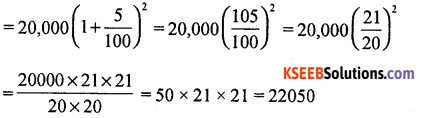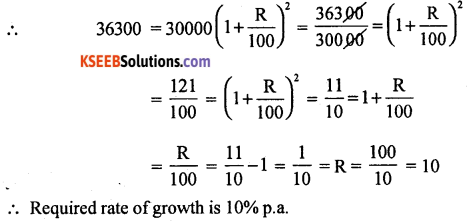# 1st PUC Basic Maths Question Bank Chapter 8 Simple Interest and Compound Interest

Students can Download Basic Maths Chapter 8 Simple Interest, Compound Interest and Annuities Questions and Answers, Notes Pdf, 1st PUC Basic Maths Question Bank with Answers helps you to revise the complete Karnataka State Board Syllabus and score more marks in your examinations.

## Karnataka 1st PUC Basic Maths Question Bank Chapter 8 Simple Interest, and Compound Interest

Question 1.
Find simple Interest on Rs 7,300 from 15 may 2009 to 8th October 2009 at 10% p.a.
Given P = Rs.7,300, R = 10%
Time = May + June + July + Aug + Sept. + Oct.
= 16 + 30 + 31 + 31 + 30 + 8 = l46days.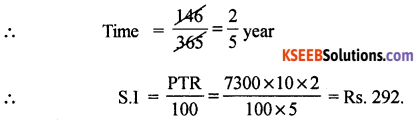Question 2.
Determine the principal which will amount to Rs. 15,000 in 4 years at 8% p.a.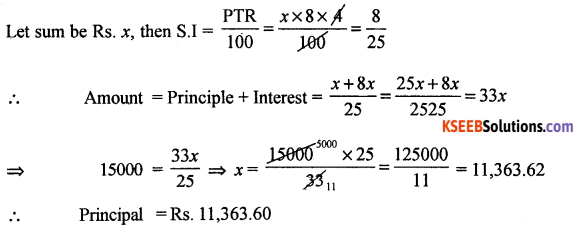Question 3.
In how many years will a sum of money double itself at 18.75% p.a simple interest.
Let the principal be Rs. P, then amount = Rs. 2P
∴ SI = Amount – PrincipIe = Rs. 2P – P = P; R = 18.75% p.a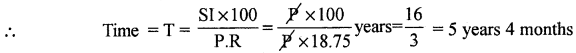Question 4.
Kumar borrowed some many at the rate of 6% p.a for the first two years, at the rate of 9% p.a. for the next three years, and at the rate of 14% p.a. for the period beyond live years. If he pays a total interest of Rs. 11,400 at the end of nine years, how much money did he borrow.
Let the sum borrowed be x. then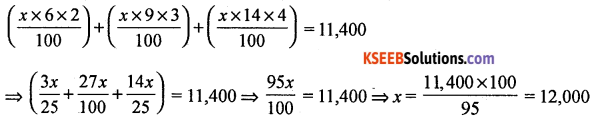Hence sum borrowed = Rs. 12,000Compound Interest

Question 1.
Find the CI amount and Cl on Rs. 12,000 for 3 years at 10% p.a. compounded annually.
P = 12,000, n = 3,R = 10%p.a
We have amount A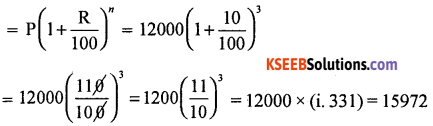∴ C.I = A.P = 15972 – 12000 = Rs. 3972

Question 2.
At what percent p.a. will a sum of Rs. 5,000 become Rs. 8,000 if the loan given for 4 years attract compound interest?
Given A = Rs. 8000, P = Rs. 5000, n = 4, R = ?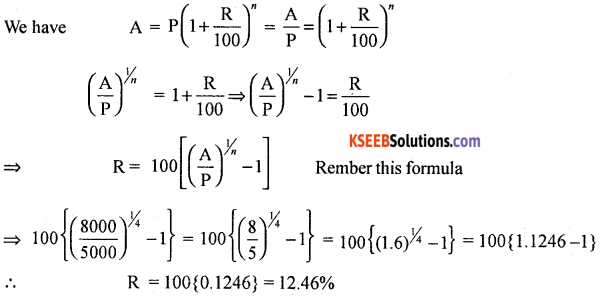Question 3.
Compute the CI. on Rs. 12,000 for 2 years at 20% p.a. when compounded half yearly.
Given P = 12,000, R = 20% p.a. and n = 2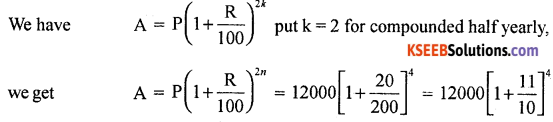= 12000$$\left(\frac{11}{10}\right)^{4}$$ = 12000 (1.4641) = 17569.20
A = Rs. 17,569.20
∴ Compound Interest = A – P = 17,569.20 – 12,000 = Rs. 5,569.20

Question 4.
A sum of money doubles itself at compound an Interest in 15 years. In how many years will it become eight times.
Let the sum of the money be Rs. P is interested at the rate of R% p.a. It is given that the moñey double it self in 15 years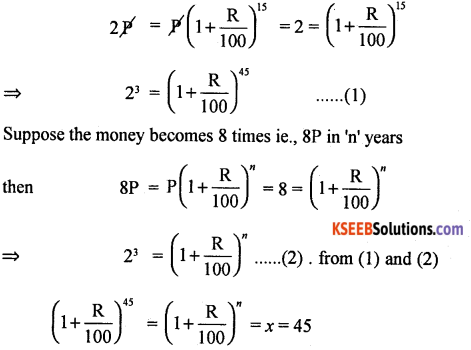∴ the money will become 8 times in 45 years.Question 5.
The population of a town in increasing at the rate of 5% p.a. What will be the population of the two as thus basic after two years if the present population is 20,0000.
We have poplulation after 2 years = $$\mathrm{p}\left(1+\frac{\mathrm{R}}{100}\right)^{n}$$